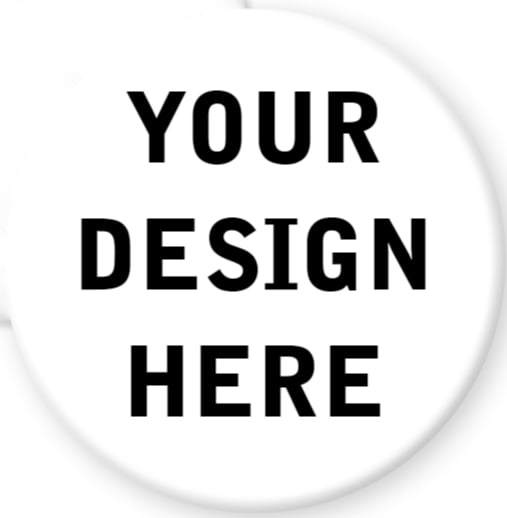# Order Custom Buttons Here

\$20.00 - \$2,400.00Custom Button Orders

Looking to make some flair for your band, zine, restaurant, brewery, hothouse, artwork, got something to say, etc...We got ya covered! If you're with a non-profit, political campaign or charity, get in touch and we'll work with your needs and budgets. Stick it to the man, with a button!

You could either send us your artwork and we'll put your design on a template for you or you could use the templates and guidelines down below and do it yourself.

All button parts and makers are Union Made in the USA.

1" pinback buttons
50=\$20
100=\$30
250=\$80
500=\$135
1000=\$220
5000=\$980
10000=\$1,700

1" magnets
50=\$35
100=\$65
250=\$165
500=\$310
1000=\$650

1.5" pinback buttons
50=\$25
100=\$40
250=\$105
500=\$195
1000=\$360
5000=\$1,550
10000=\$2,700

1.5" magnets
50=\$45
100=\$75
250=\$190
500=\$350
1000=\$650

1.5" keychains
25=\$35
50=\$65
100=\$120
250=\$295
500=\$580
1000=\$990

2.25" pinback buttons
50=\$30
100=\$45
250=\$115
500=\$220
1000=\$395
2500=\$950
5000=\$1,350
10000=\$2,400

2.25" magnets
50=\$45
100=\$80
250=\$200
500=\$380
1000=\$670

2.25" bottle openers
25=\$40
50=\$70
100=\$130
250=\$315
500=\$605
1000=\$1,030

2.25" mirror
25=\$50
50=\$90
100=\$170
250=\$325
500=\$625
1000=\$1,100

2x3" rectangle button(horizontal or vertical)
25=\$45
50=\$80
100=\$120
250=\$210
500=\$375
1000=\$640

2x3" rectangle magnet
10=\$25
25=\$50
50=\$85
75=\$110
100=\$135
125=\$160
150=\$185
175=\$210
200=\$235
225=\$260
250=\$280
500=\$525
1000=\$990

2x3" rectangle mirror
25=\$50
50=\$90
100=\$165
250=\$355
500=\$695
1000=\$1,175

If you're looking for larger quantities then listed, drop us a line for pricing at [email protected]

We review artwork to ensure you will receive the very best pinback buttons and products.

We will check your artwork to make sure it has the proper image resolution and size, art is within the safe area, includes a full bleed, and rim text(if any) is placed correctly.

If changes are needed, you will receive an email from us within 1-3 business day. If everything looks good we'll begin production.

Need help making that special button? We offer design services starting at just \$10 so drop us a line if you'd like us to help curate your designs.

• Free Ground Shipping included with every order
• Standard Turnaround: 1 week plus ground shipping time
• Need them quicker? Contact us and we'll do our best to make it happen

Please submit artwork according to the following guidelines:
• Art is in CMYK Color
• Art is at least 300 dpi
• Art is at least the size of your chosen button
• All fonts are outlined
• All linked images are embedded in file
• Fonts are 5pt size or above
• Line weight is 0.5pt thick or above

Accepted File Types (Max size: 10mb)
JPG, TIFF, PNG, GIF, BMP, SVG, PSD

Email artwork to [email protected] Please include the invoice number so we know it's your order.